Courses

# RD Sharma Solutions Ex-8.3, Lines And Angles, Class 9, Maths Class 9 Notes | EduRev

## Class 9 : RD Sharma Solutions Ex-8.3, Lines And Angles, Class 9, Maths Class 9 Notes | EduRev

The document RD Sharma Solutions Ex-8.3, Lines And Angles, Class 9, Maths Class 9 Notes | EduRev is a part of the Class 9 Course RD Sharma Solutions for Class 9 Mathematics.
All you need of Class 9 at this link: Class 9

Q 1: In the below fig, lines l1, and l2 intersect at O, forming angles as shown in the figure. If x = 45. Find the values of x, y. z and u.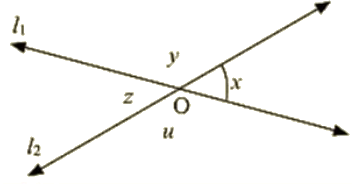Ans : Given that

X = 45°, y =?, Z = ?, u=?

Vertically opposite angles are equal

Therefore z = x = 45

z and u are angles that are a linear pair

Therefore, z + u = 180

z = 180 – u

• u = 180 – x
• u = 180 – 45
• u = 135

x and y angles are a linear pair

• x+ y = 180
• y = 180 – x
• y =180 – 45
• y = 135

Hence, x = 45, y = 135, z = 135 and u = 45

Q 2 :  In the below fig. three coplanar lines intersect at a point O , forming angles as shown in the figure. Find the values of x, y, z and u .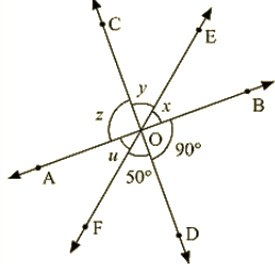Ans : Vertically opposite angles are equal

So∠SOD = z = 90

∠DOF = y = 50

Now, x + y + z = 180 [Linear pair]

• x + y + z = 180
• 90 + 50 + x = 180
• x = 180 – 140
• x = 40

Hence values of x, y, z and u are 40, 50, 90 and 40 respectively in degrees.

Q 3 : In the given fig, find the values of x, y and z.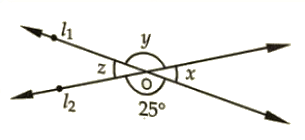Ans : From the given figure

y = 25 [Vertically opposite angles are equal]

Now∠x+∠y = 180

[Linear pair of angles]

• x = 180 – 25
• x = 155

Also,

z = x = 155 [Vertically opposite angles]

y = 25

z = 155

Q 4 : In the below fig. find the value of x ?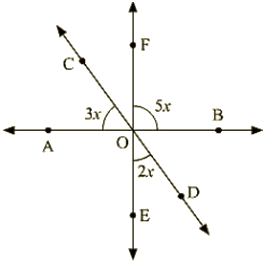Ans : Vertically opposite angles are equal

AOE = BOF = 5x

Linear pair

∠COA+∠AOE+∠EOD = 180

• 3x + 5x + 2x = 180
• 10x = 180
• x = 180/10
• x = 18

Hence, the value of x = 18

Q 5 : Prove that bisectors of a pair of vertically opposite angles are in the same straight line.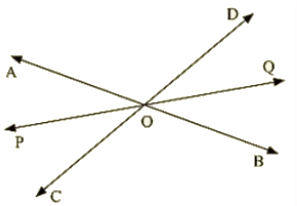Ans : Given,

Lines A0B and COD intersect at point O, such that

/AOC – /BOO

Also OE is the bisector of ADC and OF is the bisector of BOD

To prove: EOF is a straight line, vertically opposite angles are equal

AOD = BOC = 5x —–(1)

Also ,

AOC +BOD

• 2 AOD = 2 DOF —–(2)

We know,

Sum of the angles around a point is 360

• 2 AOD + 2 AOE + 2 DOF = 360
• AOD + AOE + DOF = 180

From this we can conclude that EOF is a straight line.

Given that: – AB and CD intersect each other at O

OE bisects COB

To prove: AOF = DOF

Proof: OE bisects COB

COE = EOB = x

Vertically opposite angles are equal

• BOE = AOF = x —– (1)
• COE = DOF= x —– (2)

From (1) and (2),

∠AOF=∠DOF=x

Hence Proved.

Q 6 : If two straight lines intersect each other, prove that the ray opposite to the bisector of one of the angles thus formed bisects the vertically opposite angle.

Ans : Let AB and CD intersect at a point O

Also, let us draw the bisector OP of AOC

Therefore,

AOP = POC —–(i)

Also, let’s extend OP to Q.

We need to show that, OQ bisects BOD

Let us assume that OQ bisects BOD , now we shall prove that POQ is a line.

We know that,

AOC and DOB are vertically opposite angles. Therefore, these must be equal,

that is : AOC = DOB ` —–(ii)

AOP and BOQ are vertically opposite angles.

Therefore, AOP = BOQ

Similarly, POC = DOQ

We know that : AOP + AOD + DOQ + POC + BOC + BOQ = 360°

2 AOP + AOD +2 D0Q+ BOC =360°

2 AOP + 2 AOD + 2 DOQ = 360°

2( AOP + AOD + DOQ) = 360°

AOP + AOD +DOQ = 360 /2

AOP + AOD + DOQ = I 80°

Thus, POQ is a straight line.

Hence our assumption is correct. That is,

We can say that if the two straight lines intersect each other, then the ray opposite to the bisector of one of the angles thus formed bisects the vertically opposite angles.

Q 7 : If one of the four angles formed by two intersecting lines is a right angle. Then show that each of the four angles is a right angle.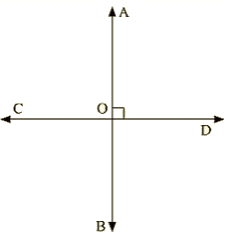Ans : Given,

AB and CD are two lines intersecting at O, such that

∠BOC = 90,∠AOC = 90∠AOD = 90° and ∠BOD = 90

{figure}

Proof :

Given that B0C = 90

Vertically opposite angles are equal

BOC = AOD = 90

A0C, BOC are a Linear pair of angles

∠AOC+∠BOC = 180

[Linear pair]

• AOC + 90 = 180
• AOC = 90

Vertically opposite angles

Therefore, AOC = BOD = 90

Hence, AOC = BOC = BOD = AOD = 90

Q 8 : In the below fig. rays AB and CD intersect at O.

(I) Determine y when x = 60

(ii) Determine x when y = 40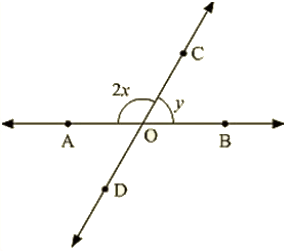Ans :  (i) Given x = 60

AOC, BOC are linear pair of angles

∠AOC+∠BOC = 180

⇒ 2x + y = 180

⇒ 2 (60)+ y = 180 [since x = 60]

⇒ y = 60

(ii) Given y = 40

AOC and BOC are linear pair of angles

∠AOC+∠BOC = 180

⇒ 2x + y = 180

⇒ 2x + 40 = 180

⇒ 2x =180 – 140

⇒ 2x = 140

⇒ x = 70

Q 9 : In the below fig. lines AB. CD and EF intersect at O. Find the measures of ∠AOC,∠COF,∠DOE and ∠BOF.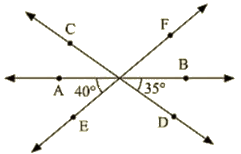Ans : AOE and EOB are linear pair of angles

∠AOE+∠EOB = 180

∠AOE+∠DOE+∠BOD = 180

⇒ DOE = 180 – 40 – 35 = 105

Vertically opposite side angles are equal

DOE = COF =105

Now,∠AOE+∠AOF = 180

[Linear pair]

AOE + AOC + COF = 180

⇒ 40 + AOC +105 = 180

⇒ AOC = 180 – 145

⇒ AOC = 35

Also, BOF = AOE = 40 (Vertically opposite angles are equal)

Q 10 : AB, CD and EF are three concurrent lines passing through the point O such that OF bisects BOD. If BOF = 35. Find BOC and AOD.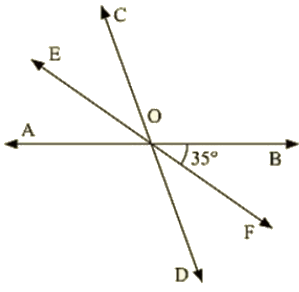Ans : Given

OF bisects BOD

BOF = 35

Angles BOC and AOD are unknown

BOD = 2 BOF = 70 [since BOD is bisected]

BOD = AOC = 70 [ BOD and AOC are vertically opposite angles]

Now,

BOC + AOC = 180

BOC +70 = 180

BOC =110

AOD = BOC = 110 (Vertically opposite angles]

Q 11 : In below figure, lines AB and CD intersect at O. If ∠AOC+∠BOE = 70 and ∠BOD = 40, find ∠BOE and reflex ∠COE?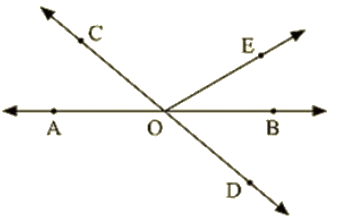Ans : Given that

AOC + BOE = 70 and BOD = 40

To find BOE

Here , BOD and AOC an vertically opposite angles

BOD = AOC = 40

Given,∠AOC+∠BOE = 70,,

⇒ 40 + BOF = 70

⇒ BOF = 70 – 40

⇒ BOE = 30

AOC and BOC are lines pair of angles

⇒ AOC + COF + BOE = 180

⇒ COE = 180 – 30 – 40

⇒ COE = 110

Hence, Reflex COE = 360 – 110 – 250.

Q 12 : Which of the following statements are true (T) and which are false (F)?

(i) Angles forming a linear pair are supplementary.

(ii) If two adjacent angles are equal and then each angle measures 90

(iii) Angles forming a linear pair can both acute angles.

(iv) If angles forming a linear pair are equal, then each of the angles have a measure of 90

Ans : (i) True

(ii) False

(iii) False

(iv) true

Q 13 : Fill in Inc blanks so as to make the following statements true:

(i) If one angle of a linear pair is acute then its other angle will be______

(ii) A ray stands on a line, then the sum of the two adjacent angles so formed is ______

(iii) If the sum of two adjacent angles is 180, then the ______ arms of the two angles are opposite rays.

Ans :

(i) Obtuse angle

(ii) 180 degrees

(iii) Uncommon

Offer running on EduRev: Apply code STAYHOME200 to get INR 200 off on our premium plan EduRev Infinity!

91 docs

,

,

,

,

,

,

,

,

,

,

,

,

,

,

,

,

,

,

,

,

,

,

,

,

,

,

,

,

,

,

;